﻿ Python: Takes two lists and returns True if they have at least one common member - w3resource# Python: Takes two lists and returns True if they have at least one common member

## Python List: Exercise-11 with Solution

Write a Python function that takes two lists and returns True if they have at least one common member.

Example - 1 :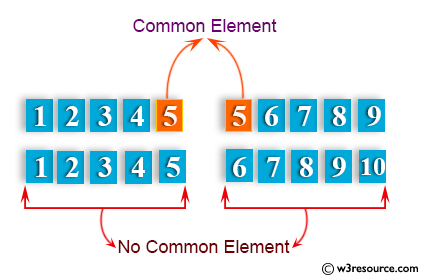Example - 2 :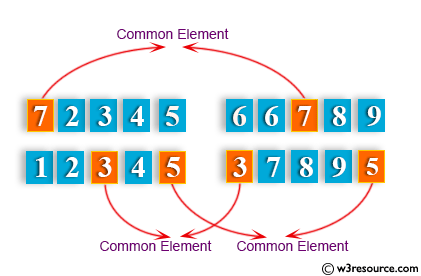Example - 3 :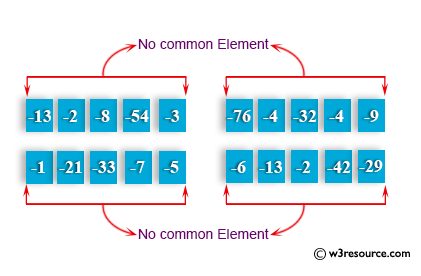Sample Solution-1:

Python Code:

``````def common_data(list1, list2):
result = False
for x in list1:
for y in list2:
if x == y:
result = True
return result
print(common_data([1,2,3,4,5], [5,6,7,8,9]))
print(common_data([1,2,3,4,5], [6,7,8,9]))
```
```

Sample Output:

```True
None
```

Flowchart: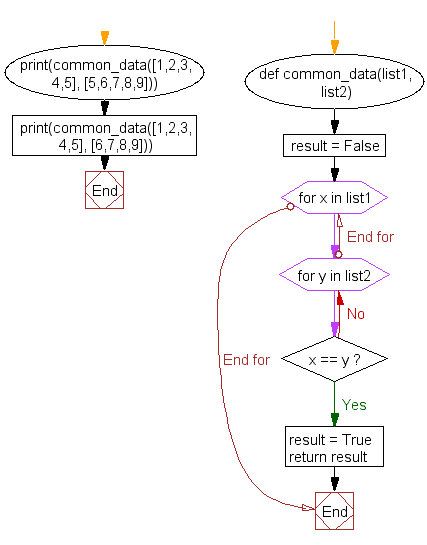## Visualize Python code execution:

The following tool visualize what the computer is doing step-by-step as it executes the said program:

Sample Solution-2:

• Check if any value in lsts is contained in nums using a for loop.
• Return True if any one value is found, False otherwise.

Python Code:

``````def test_includes_any(nums, lsts):
for x in lsts:
if x in nums:
return True
return False
print(test_includes_any([10, 20, 30, 40, 50, 60], [22, 42]))
print(test_includes_any([10, 20, 30, 40, 50, 60], [20, 80]))
```
```

Sample Output:

```False
True
```

Flowchart: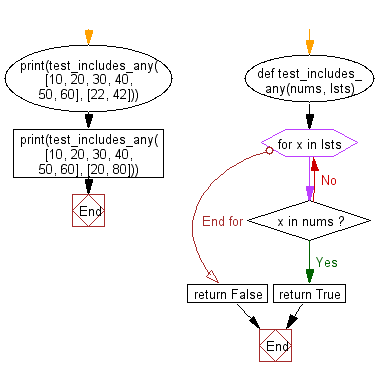## Visualize Python code execution:

The following tool visualize what the computer is doing step-by-step as it executes the said program:

Python Code Editor:

Have another way to solve this solution? Contribute your code (and comments) through Disqus.

What is the difficulty level of this exercise?

Test your Python skills with w3resource's quiz

﻿

## Python: Tips of the Day

```print(2_000_000)
```2000000